# Number Lines 0 20 PrintableUpdated on Nov 24, 2022By Printablee Team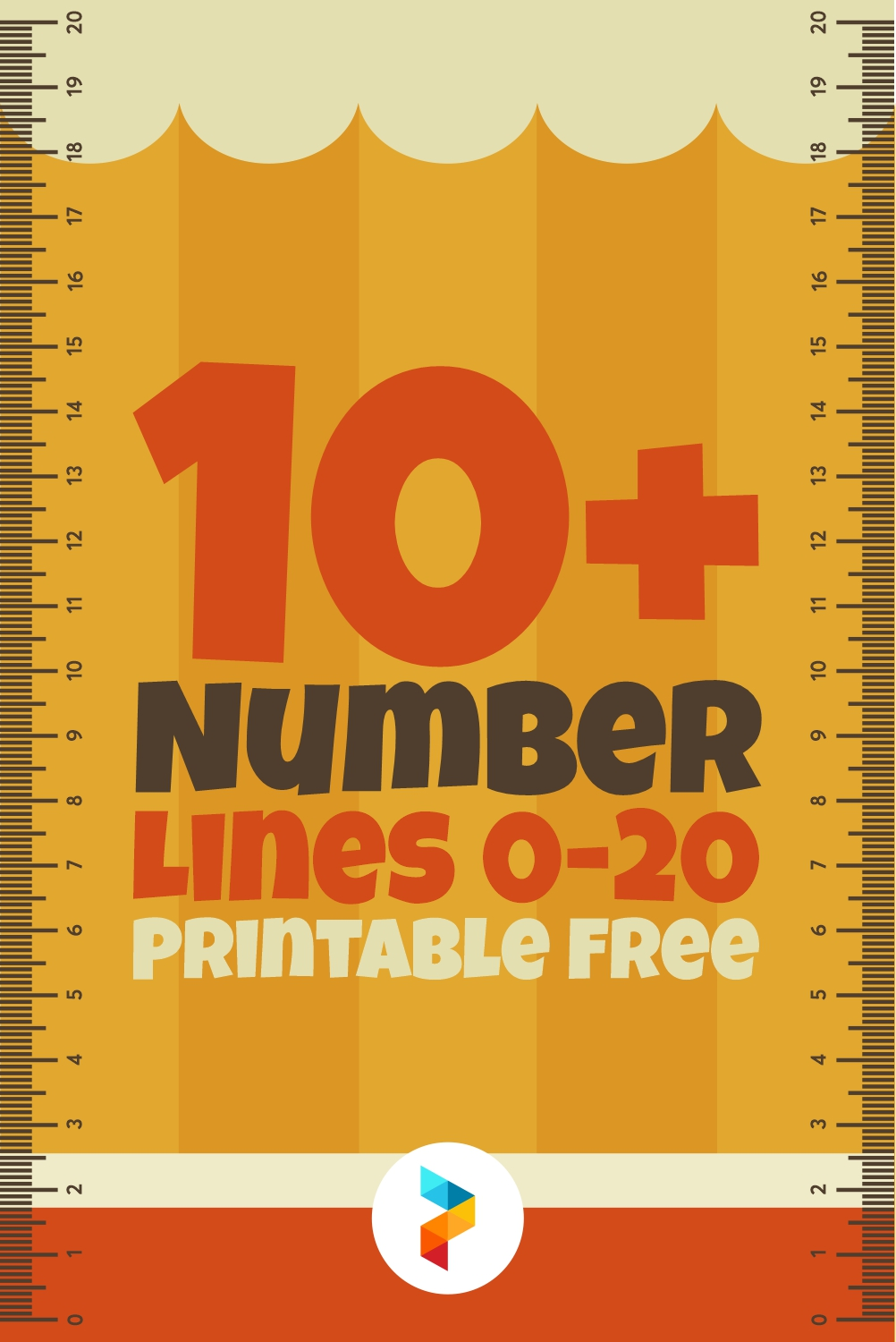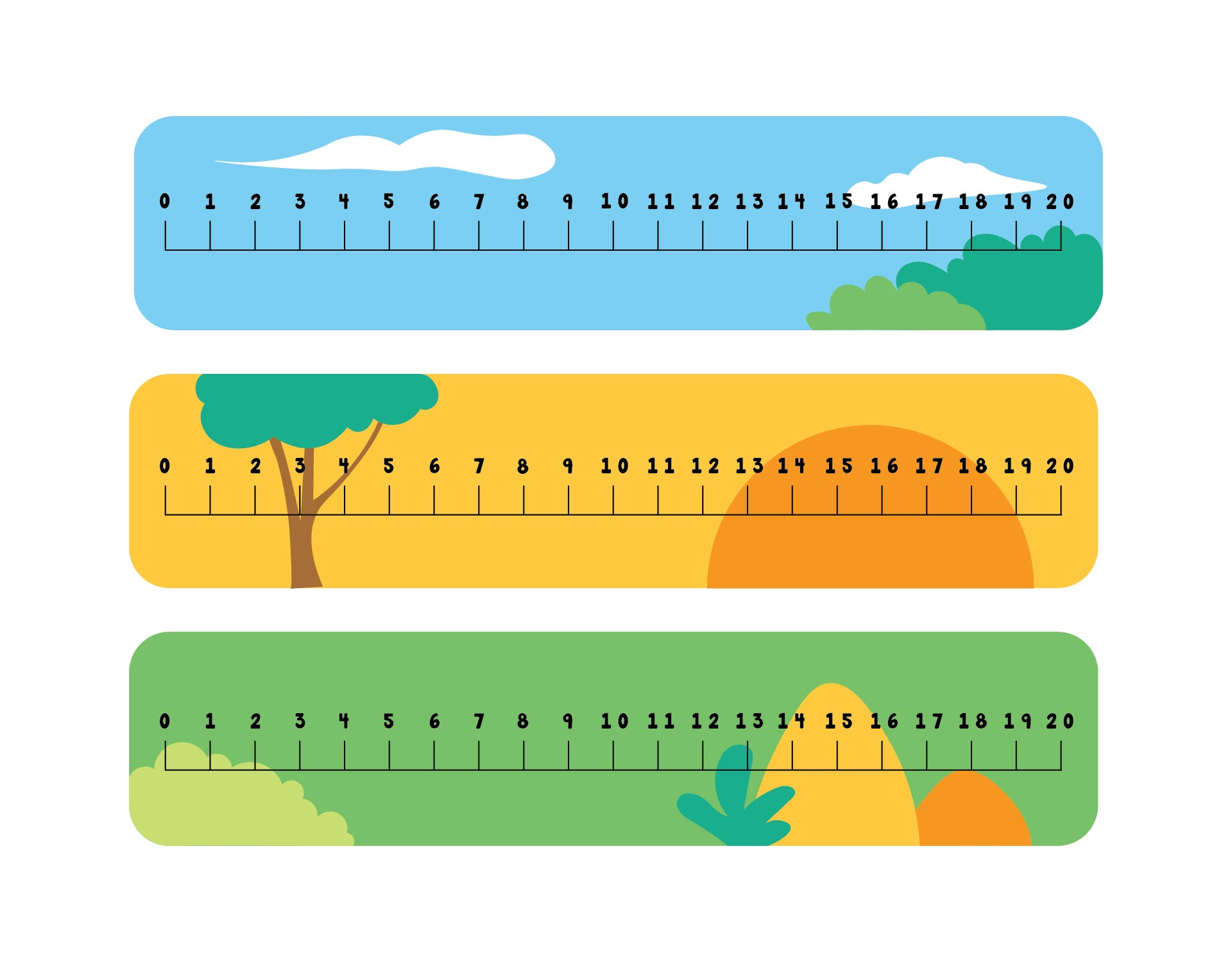## What are Number Lines?

Number lines are a way to visually represent numbers by using horizontal lines. So, number lines are horizontal lines that are straight and there are several numbers on that line. Those numbers are placed at certain distances along that line. Well, there is a starting point and an ending point for the horizontal line.

The line is sometimes referred to as a closed number line. Meanwhile, the number line can be empty. So, it doesn't have to be filled with numbers.

In addition to horizontal lines, number lines can also be vertical lines. Well, number lines with vertical lines are called number ladders.

Number lines are actually taught to elementary school children. However, number lines can be taught in kindergarten. What exactly is the function of the number lines? So, number lines are used to improve children's skills in counting such as addition, subtraction, and various other calculations.

Not only for students, but number lines also help teachers and parents teach numbers and math calculations to children. Number lines are also a great tool to provide feedback and two-way communication from teacher to student or from student to teacher.

So, if children have difficulty doing calculations, you should implement the number lines method. The reason is, number lines are easier to read and understand. So, children can learn about numbers and calculations through these lines. It will be easier for them because visual number recognition is more effective for kids.

## What is the Basic Concept of Number Lines?

There are several things that become the basic concept of number lines. This concept is also the main rule when using the number lines.

• Numbers can increase from left to right.
• Numbers will decrease if moving from right to left.
• There is a space or distance between the numbers in one line. So, the value between the intervals on the line is the same.
• Number lines can contain various numbers such as fractions, positive numbers, negative numbers, and decimal numbers.

## How to Use Number Lines for Kids?

The use of number lines depends on the age and school level of the children. So, the number lines for preschoolers with elementary school kids will be different. Then, how to use number lines as early numeracy learning according to the age and educational level of the children?

• Kindergarten: For kindergarten, number lines can help children learn to count up to 100. Make a number line from 1-10 and 1-20. The teacher can teach about the shape of the line, the numbers, and the distance between the numbers on the line.
• Grade 1: Meanwhile, grade 1 students can learn to add and subtract using number lines. Ask the children to make number lines with the numbers 1-20. Then, give them addition and subtraction problems. The children have to count them using the number lines.
• Grade 2: Grade 2 students can already be taught about calculating hundreds by using the number lines. However, the arithmetic operations that are still used are addition and subtraction.
• Grade 3: Well, grade 3 students can already use number lines to do multiplication and division calculations. However, use some numbers in the number lines so children understand how to use them first.

Meanwhile, for children in grades 4 and above, number lines can be used to calculate various mathematical operations such as addition, subtraction, multiplication, division, and combinations of these various calculation operations.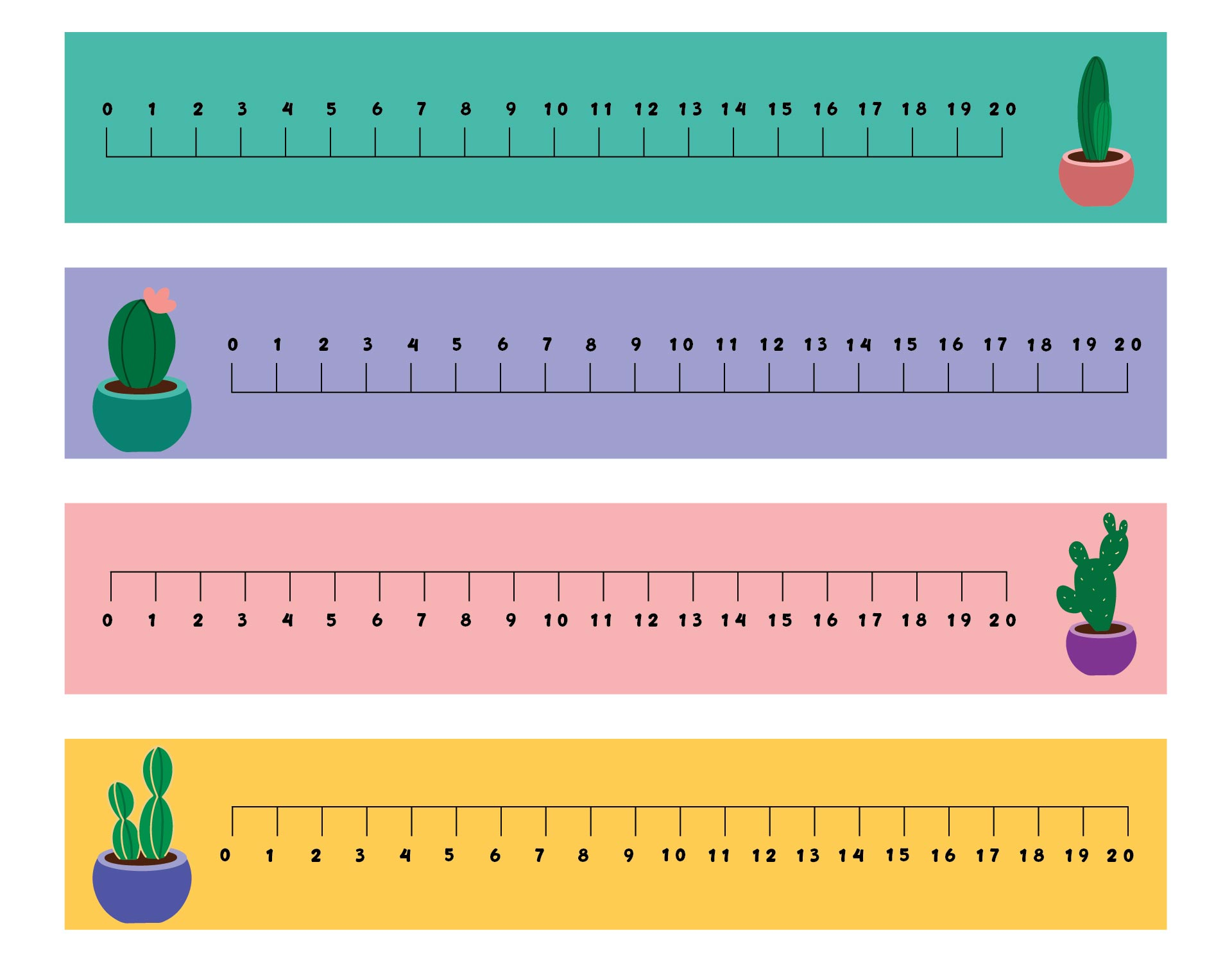We also have more printable number you may like:
Printable Number Line 1-30
Printable Number Line 1-20
12 Printable Number Worksheets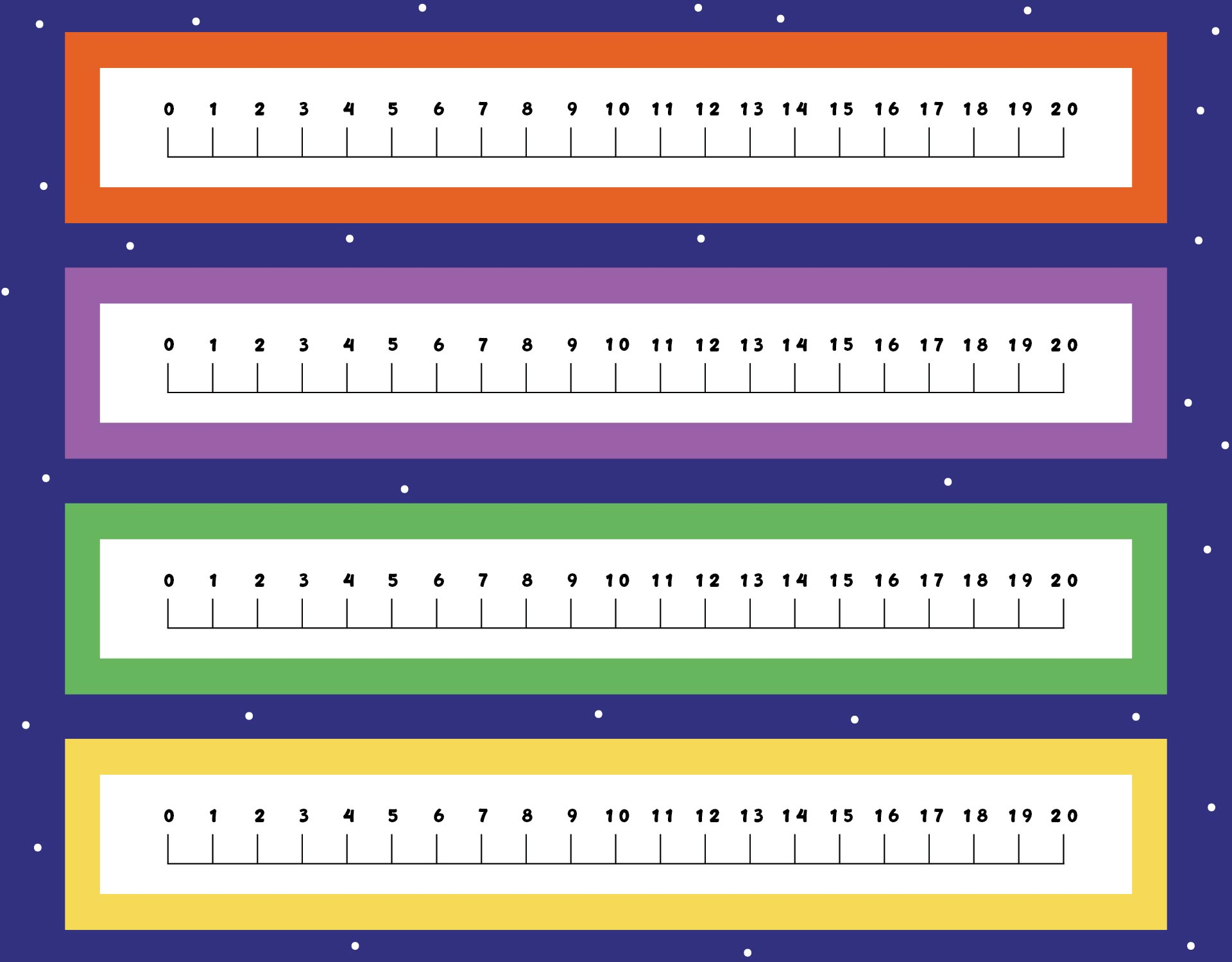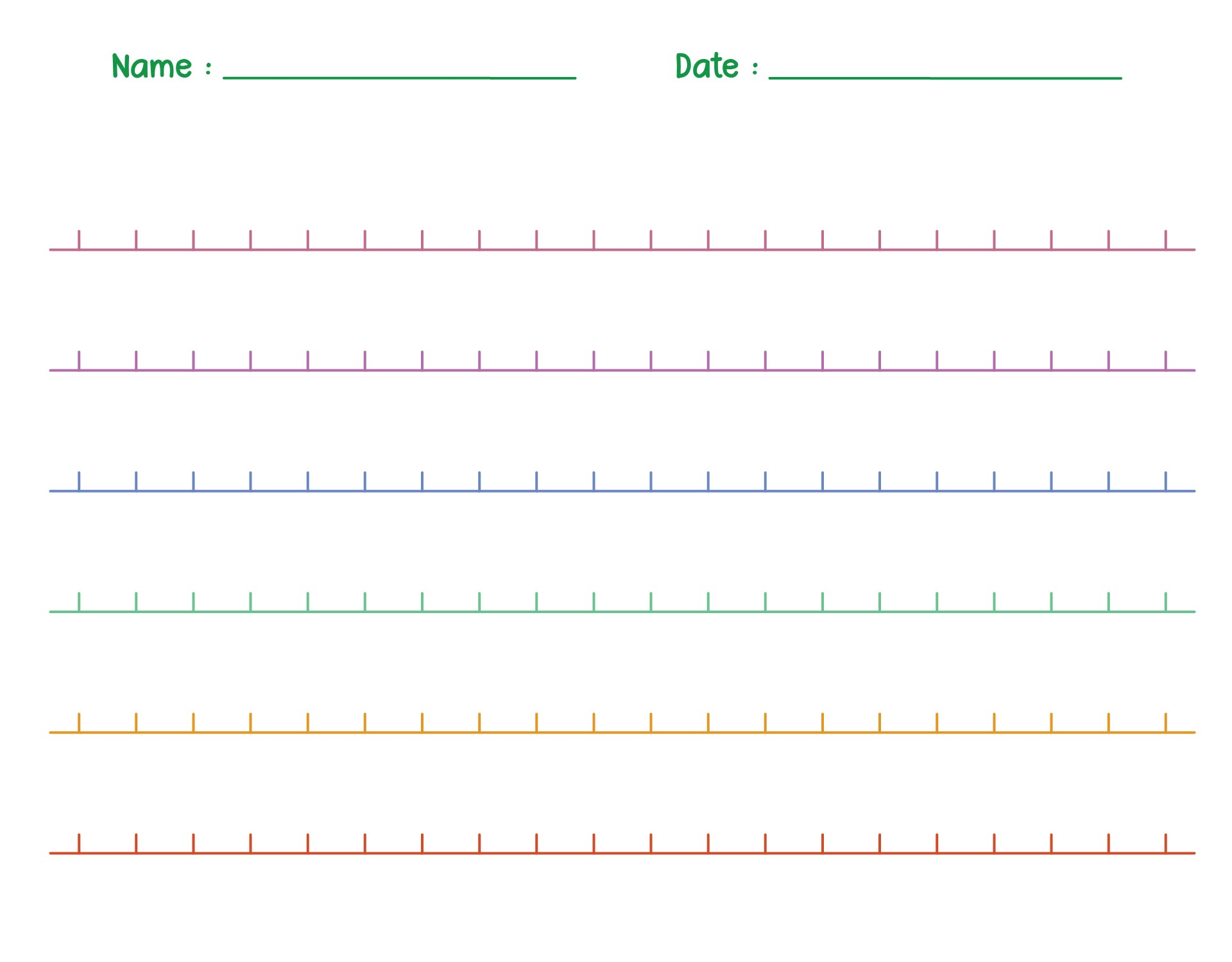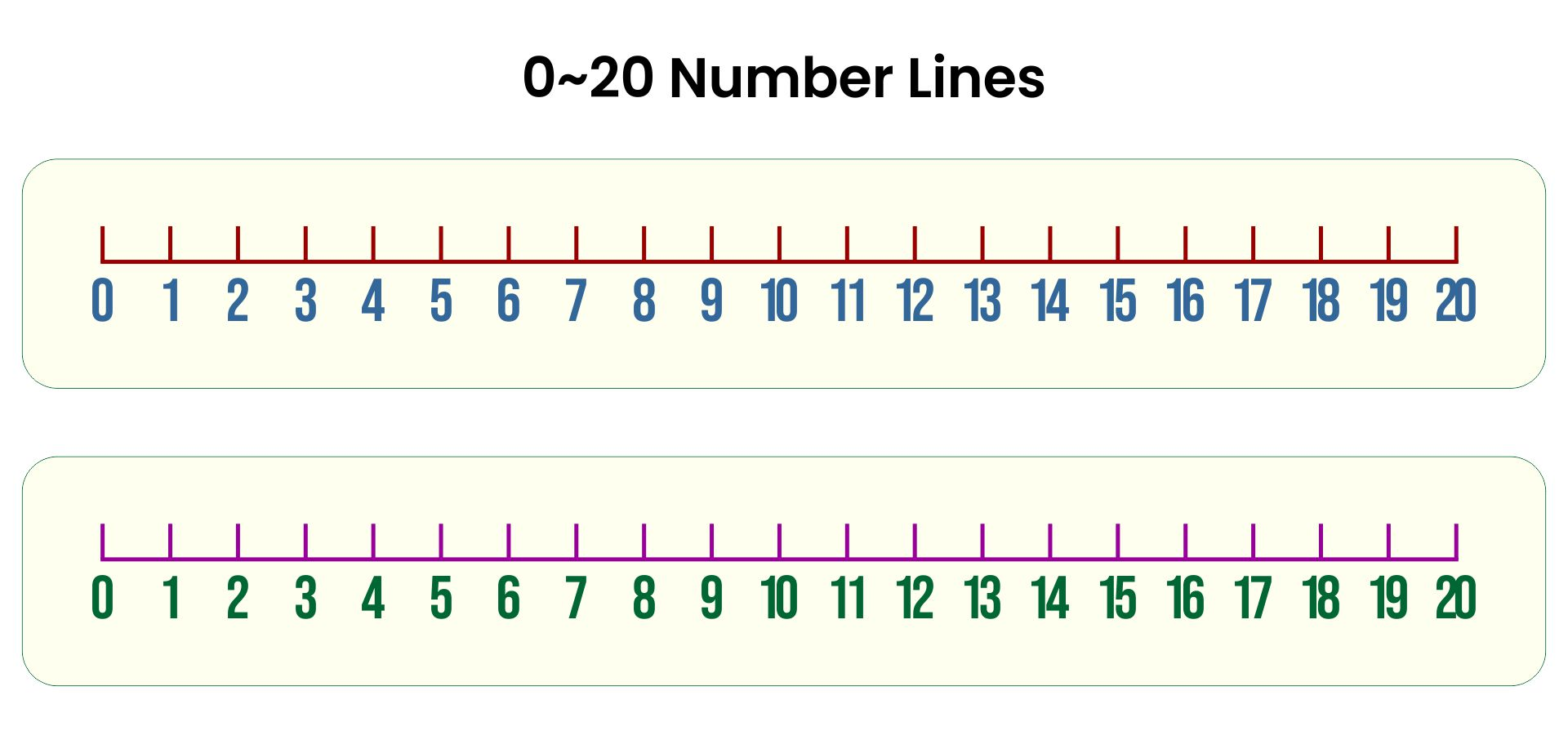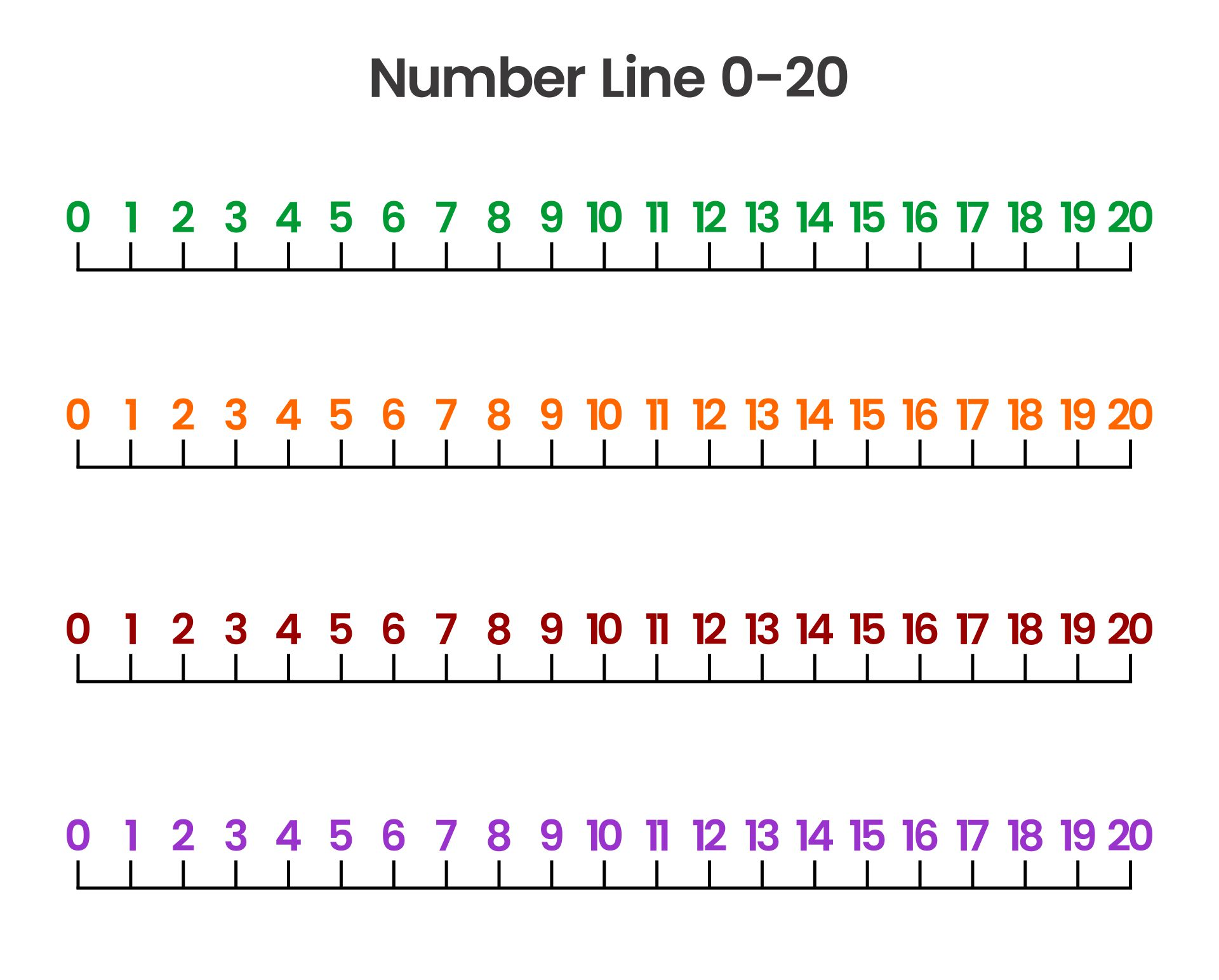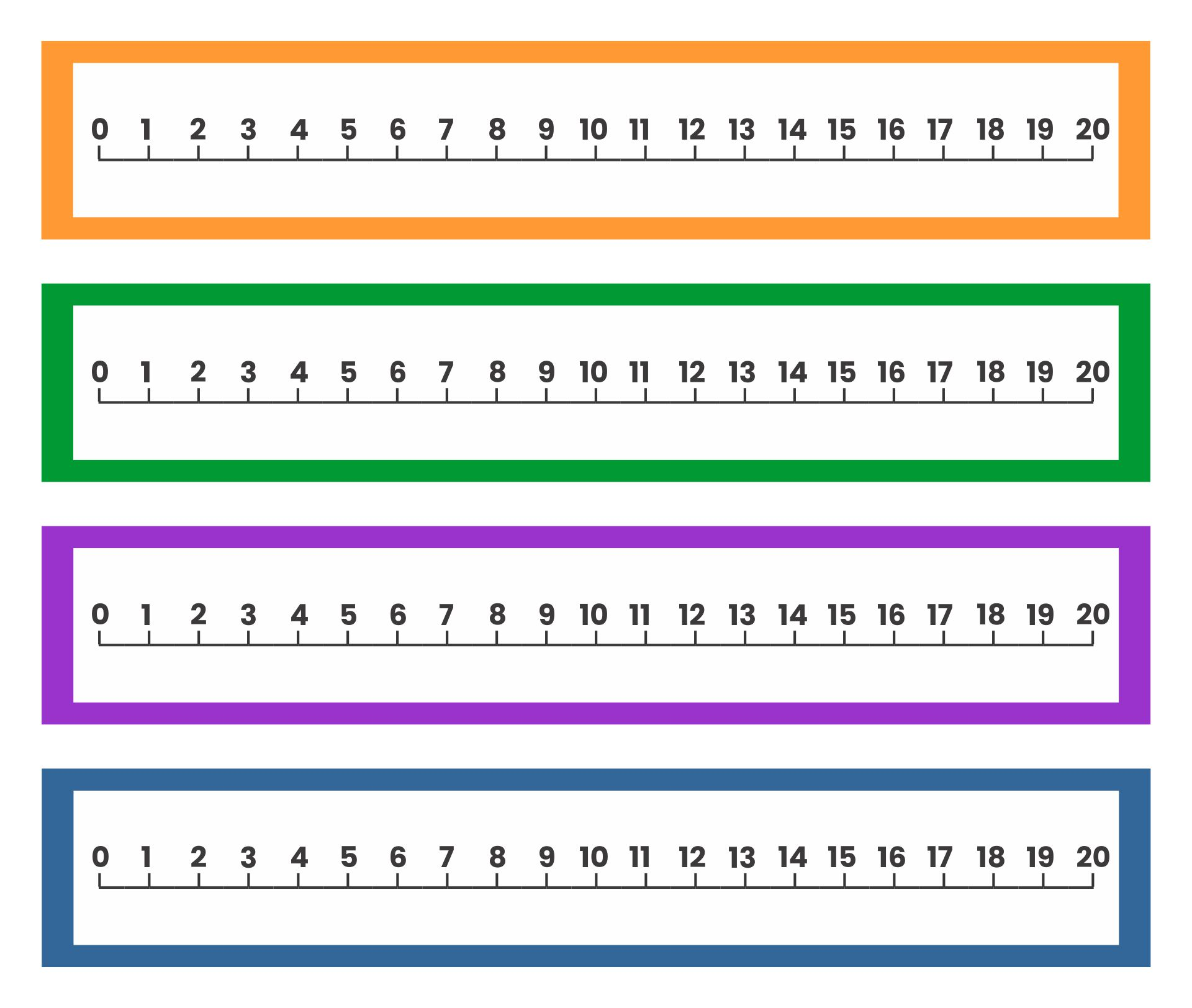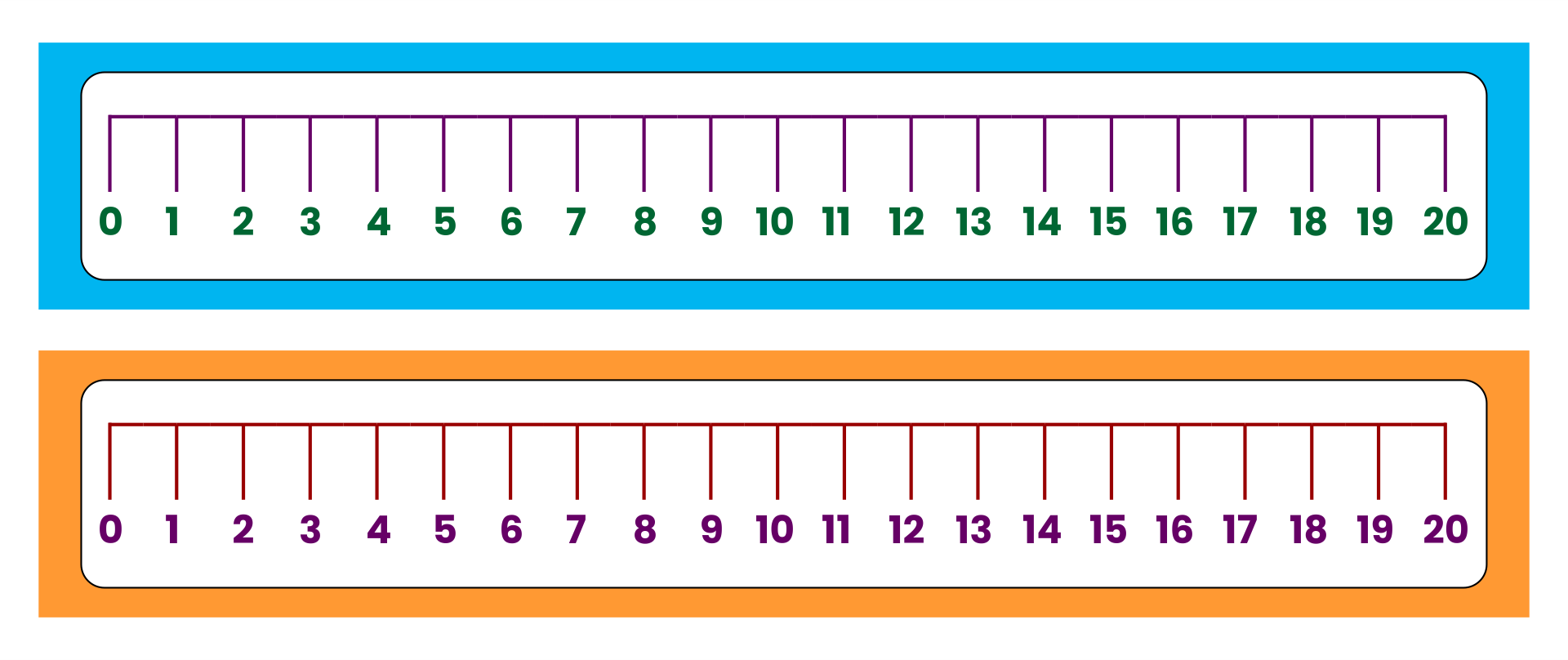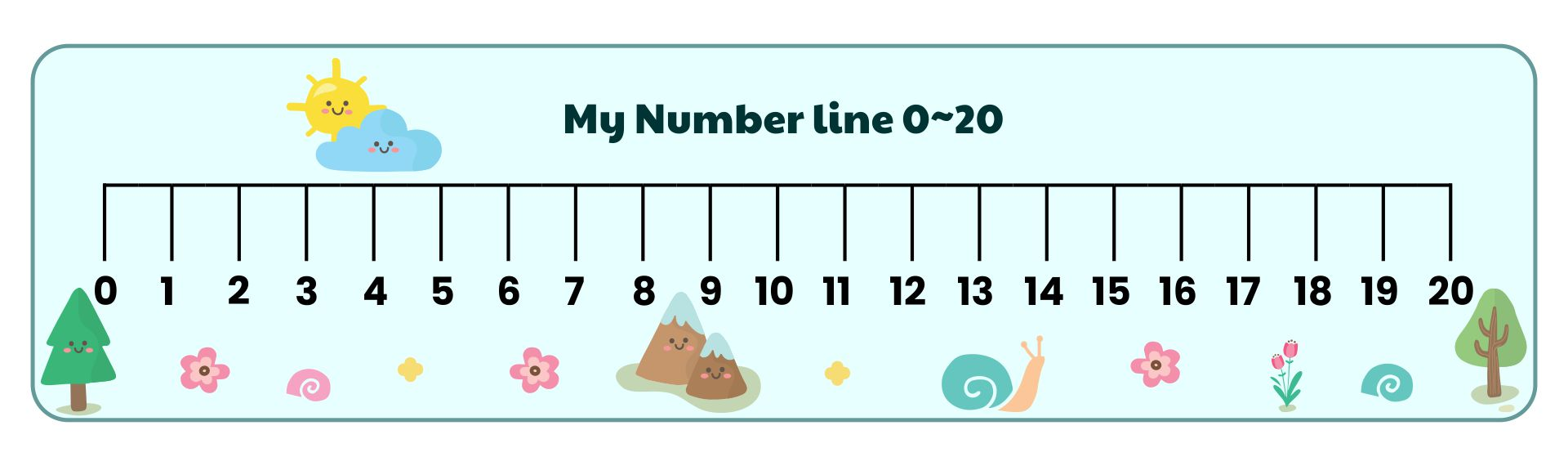## What are the Number of Line Activities for Children?

To make children able to understand number lines correctly, you need to make several number line activities for children. Here are some of the best number line activities that you can teach to your kids.

• The first activity you can do is make lines on the floor using crayons. After that, place some toys on the line. Don't forget to write down the numbers on each of these toys. Well, children can learn to count using the number lines.

• In addition, you can also have dotted number lines. The trick is to make horizontal lines on paper. Then, write down a number from 0-10. So, ask the children to draw a dot according to the number written on it. An example is the number 1. Children must draw 1 dot under the number.

• If you have Lego at home, you can use it for the number lines activity. Arrange the Lego into a long line shape. Then, write the numbers on the Lego using a pen.

• You also make clothespins as a tool to help children use number lines. Don't forget to write down the numbers on the clothespins. After that, attach the clothespins to the rope.

• Are there dominoes in your house? So, you can use these dominoes to do a number line activity with the children. The method is very easy. You only need to write a few numbers on a piece of paper. Then, arrange the papers on the floor. Next, the children must place the number of dominoes according to the number written on the paper.

• You can make number line crafts with children. Use woolen thread and cut it. After that, stick the woolen thread on a piece of paper using glue. Next, write a number next to the woolen thread. Just use numbers 1-10 and teach children to count using the number lines. Don't forget to ask the children to decorate the number lines to make them look more attractive.